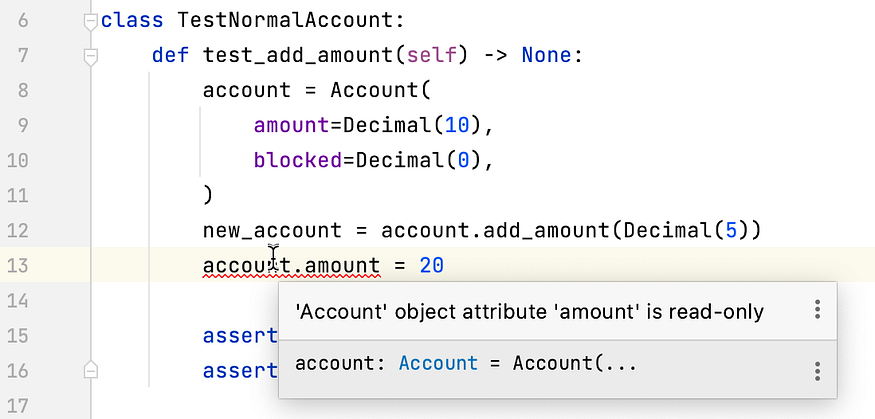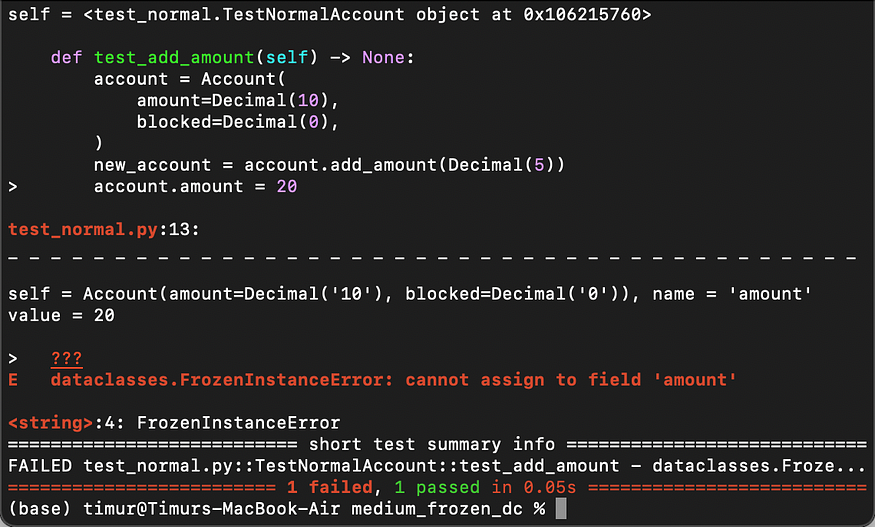# Why and How to Write Frozen Dataclasses in Python

## The difference between frozen and non-frozen dataclasses.The philosophy behind the frozen classes is pretty interesting.

We will start with an example of a normal, non-frozen, dataclass in Python that represents a Bank Account. Then we will transform it into a frozen class and discuss the difference.

### A Normal Mutable Dataclass

So, we create a Bank Account so that we can add or block some amount. It is extremely simple and there is no need to explain it:

``````from dataclasses import dataclass
from decimal import Decimal

@dataclass
class Account:
amount: Decimal
blocked: Decimal

def add_amount(self, amount: Decimal) -> None:
self.amount += amount

def block_amount(self, amount: Decimal) -> None:
if amount > self.amount:
raise ValueError("Insufficient balance")
self.blocked += amount

@property
def available_amount(self) -> Decimal:
return self.amount - self.blocked
``````

Now, we want to test it to be sure everything works fine:

``````import pytest
from decimal import Decimal
from main import Account

class TestNormalAccount:
account = Account(
amount=Decimal(10),
blocked=Decimal(0),
)
assert account.available_amount == 15

def test_block_amount(self) -> None:
account = Account(
amount=Decimal(10),
blocked=Decimal(0),
)
with pytest.raises(ValueError):
account.block_amount(Decimal(11))
assert account.available_amount == 10
account.block_amount(Decimal(7))
assert account.available_amount == 3
``````

And we can see that the tests are green:

``````============================= test session starts collected 2 items
test_normal.py ..                                                        [100%]
============================== 2 passed in 0.01s
``````

### Transformation to a Frozen Dataclass

What can be simpler than this? Simply add the “frozen=True” to the decorator:

``````@dataclass(frozen=True)
``````

and run the tests again. You will see this error:

``````E   dataclasses.FrozenInstanceError: cannot assign to field 'blocked'
``````

The problem (or the feature) is that you may not change the fields of the Account object anymore.

But how do we change it then, for sure we want it to be changed at some point.

The idea is that the functions that mutate the object should now return new objects instead. If you have worked with Pandas Series before, then you may remember that any modifications return new objects instead of mutating. For example, let's look at the reshape function:

``````a = a.reshape((2,3))
``````

and not just:

``````a.reshape((2,3))
``````

We apply the same principle: we do not change the object itself, but we return a similar but new object.

``````import pytest
from decimal import Decimal
from main import Account

class TestNormalAccount:
account = Account(
amount=Decimal(10),
blocked=Decimal(0),
)

assert new_account.available_amount == 15
assert account.available_amount == 10

def test_block_amount(self) -> None:
account = Account(
amount=Decimal(10),
blocked=Decimal(0),
)
with pytest.raises(ValueError):
account.block_amount(Decimal(11))
assert account.available_amount == 10
new_account = account.block_amount(Decimal(7))
assert new_account.available_amount == 3
assert account.available_amount == 10
``````

Here we test that the new object is updated, and the old one stays the same, i.e. with the original amount.

Now, there are two ways to return a mutated object.

The first, the naive one, is to return an absolutely new object like this:

``````import dataclasses
from dataclasses import dataclass
from decimal import Decimal

@dataclass(frozen=True)
class Account:
amount: Decimal
blocked: Decimal

def add_amount(self, amount: Decimal) -> "Account":
return Account(
amount=self.amount + amount,
blocked=self.blocked,
)

def block_amount(self, amount: Decimal) -> "Account":
if amount > self.amount:
raise ValueError("Insufficient balance")
return Account(
amount=self.amount,
blocked=self.blocked + amount,
)

@property
def available_amount(self) -> Decimal:
return self.amount - self.blocked
``````

But if there are too many fields, we do not want to duplicate all fields all the time, so we use the “dataclasses.replace” function as follows:

``````import dataclasses
from dataclasses import dataclass
from decimal import Decimal

@dataclass(frozen=True)
class Account:
amount: Decimal
blocked: Decimal

def add_amount(self, amount: Decimal) -> "Account":
return dataclasses.replace(self, amount=self.amount+amount)

def block_amount(self, amount: Decimal) -> "Account":
if amount > self.amount:
raise ValueError("Insufficient balance")
return dataclasses.replace(self, blocked=self.blocked+amount)

@property
def available_amount(self) -> Decimal:
return self.amount - self.blocked
``````

### Why?

Why should anyone write frozen classes? This only seems more complicated, doesn't it?

The main reason for this is to make sure your objects are only modified using the functions. So, your objects are truly immutable.

Let's try change the amount, for example:PyCharm immediately reacts with an error. Even if you ignore this and run the test, you will get an error:So if you have some strict logic and want to avoid any kind of object smodifications by simply changing the variables, then the frozen dataclasses should be your choice.

### Is it truly immutable?

In Python, it's more a philosophy behind immutability, private and protected variables etc. So, it's more like “i would like these methods to be private”, but doesn't make it impossible to really call a private method from outside.

Same here. You may still change the variables in the object by using something like this:

``````object.__setattr__(account, "amount", 20)
``````

Liked it? Have a look at my other articles on Python and Django. For example, you may like this one.